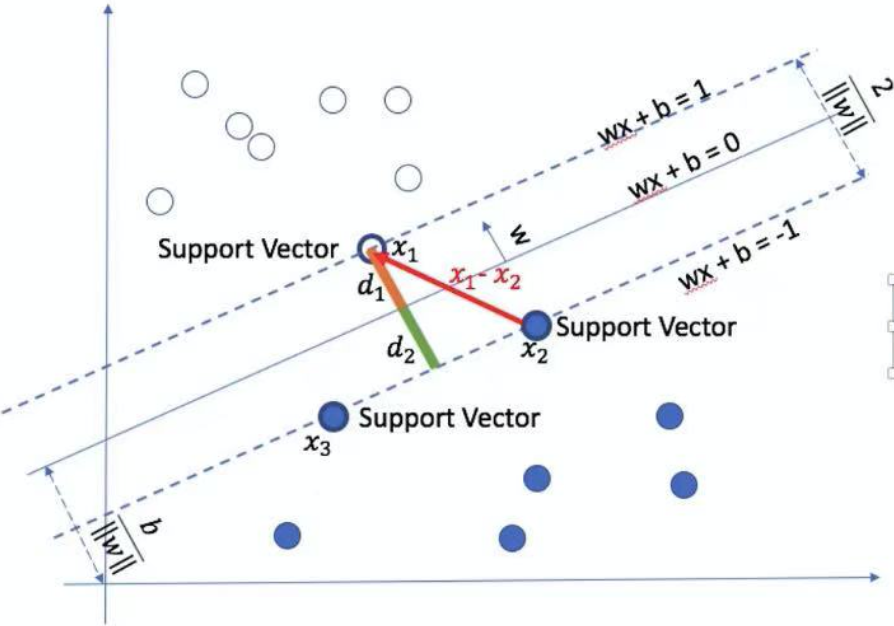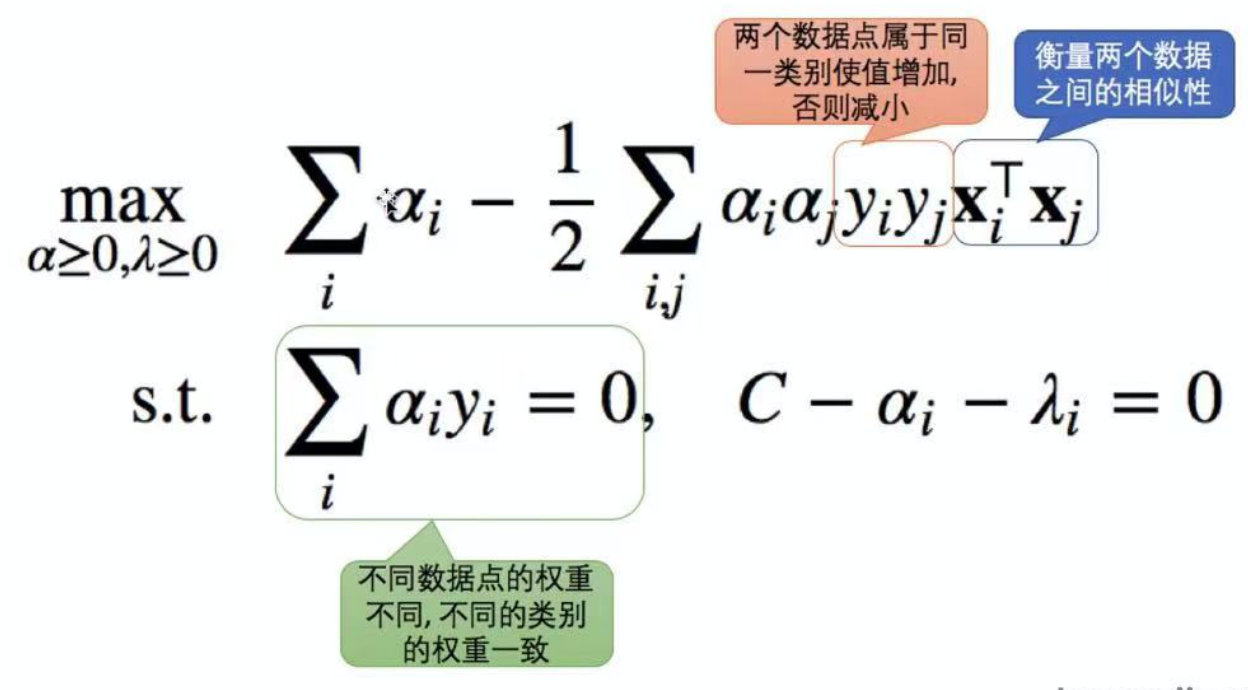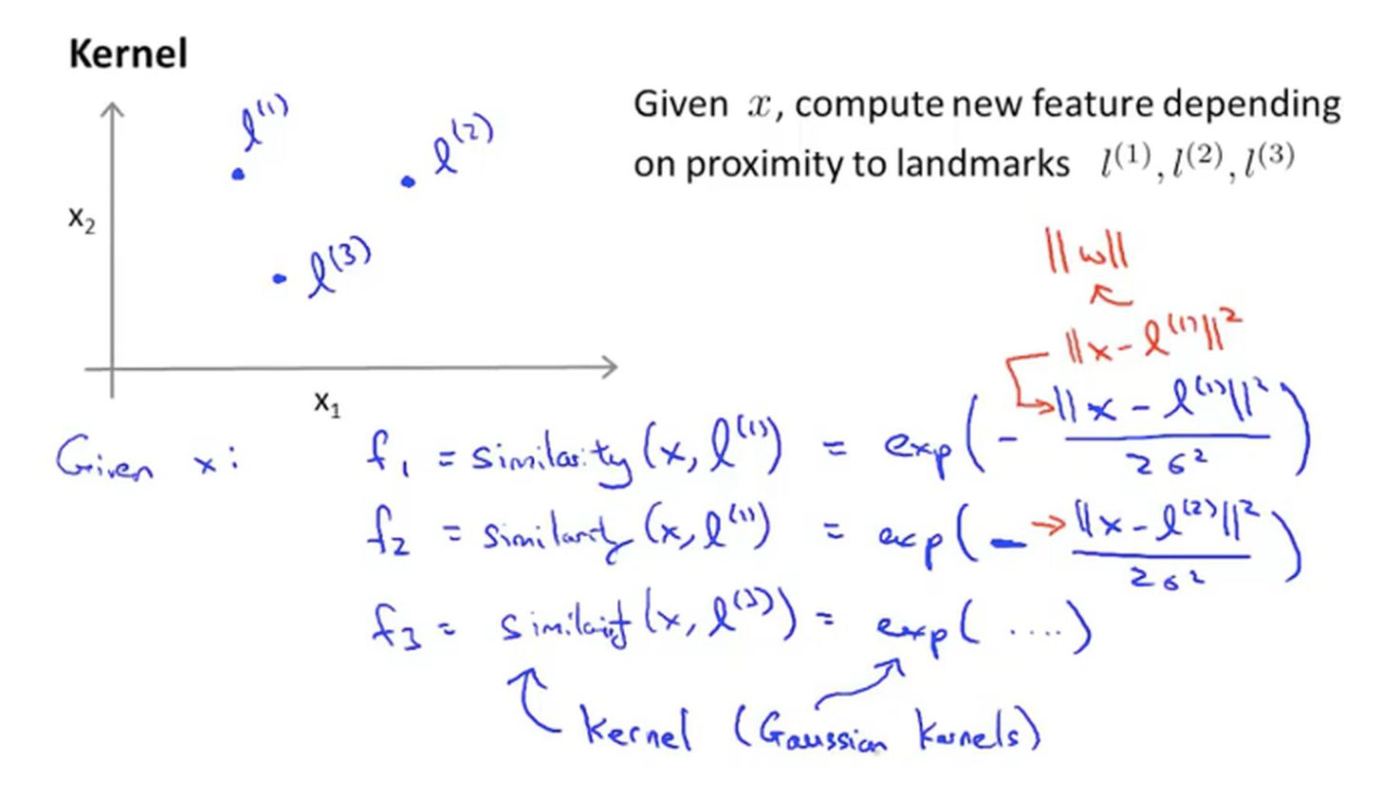# 几何定义• 假设落在上边界和下边界的两个点分别为$x_1$和$x_2$
• $w^{T} x_{1}+b=1$
• $w^{T} x_{2}+b=-1$
• $\left(w^{T} x_{1}+b\right)-\left(w^{T} x_{2}+b\right)=2$
• $w^{T}\left(x_{1}-x_{2}\right)=2$
• $d_{1}=d_{2}=\frac{w^{T}\left(x_{1}-x_{2}\right)}{2|w|_{2}}=\frac{2}{2|w|_{2}}=\frac{1}{|w|_{2}}=\frac{\frac{w^{T}}{|w|_{2}}\left(x_{1}-x_{2}\right)}{2}$
• 它的物理意义是上图中$x_1$、$x_2$的连线（即红色线）在$w$法向量上的投影向量的长度
• $d_{1}+d_{2}=\frac{2}{|w|_{2}}$

# 目标函数

## Hard Constraint

Maximize: $\frac{2}{||w||}$，且在$y_i=1$时$w^Tx_i+b\ge 1$，$y_i=-1$时$w^Tx_i+b\le -1$，如下图所示（-1和1是两个决策边界，我们希望所有点落在决策边界的两侧，不允许点出现在两个决策边界中间）：## Soft Constraint

Hard Constraint容易产生过拟合问题，如下图（圆圈圈住的是误差点，右侧的线是hard constaint，但显然左侧是我们想要找到的分界线，因为可能会有很多粉色的点落在右侧线的右边）：所以，我们允许一些点落在决策边界中间，只要给这些点加一些惩罚就好，这种就叫“Soft Constraint”：Minimize $||w||^2$ s.t. $(w^Tx_i+b)y_i\ge 1-\xi_i,\xi_i\ge0$，其中$\ni_i$表示可以允许分类起犯多大的错误，那么为了惩罚这个错误，我们把目标函数稍加修改：Minimize $||w||^2+\lambda\sum^n_{i=1}\xi_i$ s.t. $(w^Tx_i+b)y_i\ge 1-\xi_i,\xi_i\ge0$，其中$\lambda$起到平衡关系，当$\lambda$为正无穷时，相当于Hard Constraint，表示不允许犯任何错误。

## Hinge Loss

• 由$y_{i}\left(\mathbf{w}^{\top} \mathbf{x}_{i}+b\right) \geq 1-\xi_{i}$可得到$\xi_{i} \geq 1-y_{i}\left(\mathrm{w}^{\top} \mathbf{x}_{i}+b\right)$

• 由于$\xi_{i} \geq 0$可得到$\xi_{i}=\max \left(0,1-y_{i}\left(\mathbf{w}^{\top} \mathbf{x}_{i}+b\right)\right)$，这个式子就叫Hinge Loss，其函数图像如下图：由上图我们可以发现Hinge Loss的几个特点：

• Convex 凸函数，容易优化
• 在自变量小于0的部分梯度比较小（恒等于-1），对错误分类的惩罚比较轻
• 对比logistic loss，在小于0时指数级增长，损失会变得特别大
• 在自变量大于等于1的部分，值为0；只要对某个数据分类是正确的，并且正确的可能性足够高，那么就永不着针对这个数据进一步优化了
• 对比logistic loss，在大于等于1的时候还是有一些loss的
• 在自变量等于0处不可导，需要分段求导
• 在求解最优化时，只有支持向量会参与确定分界线，而且支持向量的个数远小于训练数据的个数
• 对于Hard Constraint的SVM来说，只关心支持向量的大小（在分界线上的点），对于其他点不关心
• 对于Soft Constraint的SVM来说，即关心支持向量，也关心异常点的误差

【注】：在吴恩达的机器学习课程中，认为SVM是在logistic regression的基础上，修改了损失函数定义得来的(具体可参考https://zhuanlan.zhihu.com/p/74764135)：![img](https://xiangzaixiansheng.oss-cn-beijing.aliyuncs.com/majing_blog/SVM/SVM/5.png)

也就是说，在逻辑回归中，损失函数定义为：

$J(\theta)=\frac{1}{m} \Sigma_{i=1}^{m}\left[-y^{(i)} \log \left(h_{\theta}\left(x^{(i)}\right)\right)-\left(1-y^{(i)}\right) \log \left(1-h_{\theta}\left(x^{(i)}\right)\right)\right]+\frac{\lambda}{2 m} \Sigma_{i=1}^{n} \theta_{j}^{2}$

在SVM中将损失函数定义为：$J(\theta)=C * \Sigma_{i=1}^{m}\left[y^{(i)} \operatorname{cost}_{1}\left(\theta^{T} x^{(i)}\right)+\left(1-y^{(i)}\right) \operatorname{cost}_{0}\left(\theta^{T} x^{(i)}\right)\right]+\frac{1}{2} \Sigma_{i=1}^{n} \theta_{j}^{2}$

# 支持多个类别

## OVR(one versus rest)

对于K个类别的情况，训练K个SVM，第j个SVM用于判断任意条数据是属于类别j还是属于类别非j。预测的时候，具有最大值的$w^T_ix$表示给定的数据x属于类别。

比如有3个类别A、B、C，我们训练3个SVM，第一个判断是否是A类，第二个判断是否为B类，第三个判断是否为C类，我们比较3个SVM的概率，选择概率最大的类。

## OVO(one versus one)

对于K个类别的情况，训练$K(K-1)/2$个SVM，每一个SVM只用于判读任意条数据是属于K中的特定两个类别。预测的时候，使用$K(K-1)/2$个SVM做$K*(k-1)/2$次预测，使用计票的方式决定数据被分类为哪个类别的次数最多，就认为数据$x$属于此类别。

比如有3个类别A、B、C，我们训练AB、AC、BC3个SVM用于判断是AB中的哪一类、是BC中的哪一类，是AC中的哪一类，选择投票数最多的那一类。

形象解释如下图：# 求解

## 对偶问题

原问题定义为： \begin{aligned}\min _{\mathbf{w}, b, \xi \geq 0} & \frac{1}{2} \mathbf{w}^{\top}\mathbf{w}+C \sum_{i} \xi_{i} \\\text { s.t. } y_{i}\left(\mathbf{w}^{\top}\mathbf{x}_{i}+b\right) & \geq 1-\xi_{i}, \quad i=1, \ldots, n \\\xi_{i} & \geq 0\end{aligned}

将条件表达式的左侧移动到右侧可得： $1-\xi_{i} - y_{i}\left(\mathbf{w}^{\top} \mathbf{x}_{i}+b\right) \le 0$ $-\xi_i\le0$

将上面两个式子加入到原始SVM中得到： $L(\mathbf{w}, b, \xi, \alpha, \lambda)=\frac{1}{2} \mathbf{w}^{\top} \mathbf{w}+C \sum_{i} \xi_{i}+\sum_{i} \alpha_{i}\left(1-\xi_{i}-y_{i}\left(\mathbf{w}^{\top} \mathbf{x}_{i}+b\right)\right)-\sum_{i} \lambda_{i} \xi_{i}$

其中$\alpha$和$\lambda$称为拉格朗日算子，并将原先最小化问题转化为最大化问题计算目标函数对各参数的导数，可得出：

• $\frac{\partial L}{\partial b}=0 \quad \rightarrow \quad \sum_{i} \alpha_{i} y_{i}=0$
• $\frac{\partial L}{\partial \mathbf{w}}=0 \quad \rightarrow \quad \mathbf{w}=\sum_{i} \alpha_{i} y_{i} \mathbf{x}_{i}$
• $\frac{\partial L}{\partial \xi_{i}}=0 \quad \rightarrow \quad C-\alpha_{i}-\lambda_{i}=0$

代入$\mathbf{w}=\sum_{i} \alpha_{i} y_{i} \mathbf{x}_{i}$、$\sum_{i} \alpha_{i} y_{i}=0$可以得到：$L(\xi, \alpha, \lambda)=\sum_{i} \alpha_{i}-\frac{1}{2} \sum_{i, j} \alpha_{i} \alpha_{j} y_{i} y_{j} \mathbf{x}_{i}^{\top} \mathbf{x}_{j}+\sum_{i} \xi_{i}\left(C-\alpha_{i}-\lambda_{i}\right)$

再代入$C-\alpha_{i}-\lambda_{i}=0$得到对偶形式：\begin{aligned}\max _{\alpha \geq 0, \lambda \geq 0} & \sum_{i} \alpha_{i}-\frac{1}{2}\sum_{i, j} \alpha_{i} \alpha_{j} y_{i} y_{j} \mathbf{x}_{i}^{\top} \mathbf{x}_{j} \\\text {s.t. } & \sum_{i} \alpha_{i} y_{i}=0, \quad C-\alpha_{i}-\lambda_{i}=0\end{aligned}

由于$\lambda_i$唯一需要满足的条件是大于等于0，约束条件$C-\alpha_{i}-\lambda_{i}=0$也可以改为：$\alpha_i\le C$

我们怎么去理解这个表达式呢？注意不同数据点的权重是不同的，但是不同类别的权重一致，即可以有两个A类别和1个B类别的点，但是A类别点的权重和和B类别点的权重和是相等的。

## 核函数

我们可以使用核函数来替代$x^T_ix_j$：$\begin{array}{l}\max _{\alpha \geq 0} \sum_{i} \alpha_{i}-\frac{1}{2} \sum_{i, j} \alpha_{i}\alpha_{j} y_{i} y_{j} k\left(\mathbf{x}_{i}, \mathbf{x}_{j}\right) \\\text { s.t. }\sum_{i} \alpha_{i} y_{i}=0, \quad \alpha_{i} \leq C, \quad i=1, \ldots, n \\K\left(\mathbf{x}_{i}, \mathbf{x}_{j}\right)=\left\langle\Phi\left(\mathbf{x}_{i}\right),\Phi\left(\mathbf{x}_{j}\right)\right\rangle\end{array}$

### 理解核函数

我们的假设函数可能是：$\theta_{0}+\theta_{1} x_{1}+\theta_{2} x_{2}+\theta_{3} x_{1} x_{2}+\theta_{4} x_{1}^{2}+\theta_{5} x_{2}^{2}+\ldots$的形式。为了方便，我们可以用一系列新的特征值来替换模型中的每一项，譬如：$f_{1}=x_{1}, f_{2}=x_{2}, f_{3}=x_{1} x_{2}, f_{4}=x_{1}^{2}, f_{5}=x_{2}^{2}$。则假设函数便可以转化为：$h_{\theta}(x)=\theta_{0}+\theta_{1} f_{1}+\theta_{2} f_{2}+\theta_{3} f_{3}+\theta_{4} f_{4}+\theta_{5} f_{5}+\ldots$。这种方法即通过多项式模型的方式构造新特征$f_{1}, f_{2}, f_{3} \ldots f_{n}$，那么有没有其他方式来构造新特征？有，通过核函数即可完成。

我们引入地标（landmark)$l^{(1)}, l^{(2)}, l^{(3)}$，我们可以通过判断样本x和地标间的近似程度来选取新的特征$f_1,f_2,f_3$。如上图所示，特征$f_1,f_2,f_3$都可以用similarity(x,l)函数来获取，这里的similarity(x,l)函数即被称为—核函数（kernel function）,在本例中我们用核函数中的一种—高斯核函数来举例，即：$f_{i}=\operatorname{similarity}\left(x, l^{(i)}\right)=\exp \left(-\frac{\left|x-l^{(i)}\right|^{2}}{2 \sigma^{2}}\right)$。

地标的作用是什么 ？如果一个样本x距离地标距离接近/等于0，则$f_{i} \simeq \exp (-0)=1$，否则 = 0，于是我们利用样本和地标间的关系来得出了特征f的值。看上图，可以总结出几点：

• 红色顶点处，即向量 $x=\left\{\begin{array}{l}x_{1} \\x_{2}\end{array}\right\}=\left\{\begin{array}{l}3 \\5\end{array}\right\}$和向量地标向量l重合处，即距离= 0，故此时$f=1$
• 可以看见$\sigma$越大，图像越宽，同样的x样本向量，譬如$x=\left\{\begin{array}{l}x_{1} \\x_{2}\end{array}\right\}=\left\{\begin{array}{l}4 \\4\end{array}\right\}$，这个样本在图1中就会被判定为$f=0$，而在图3中则可能被判定为$f=1$，即$\sigma$会影响到最终特征值f的判断。即随着 的改变 值改变的速率受到的控制。

假设函数值>=0时预测y = 1,否则y = 0，则通过上面的高斯核函数我们可以算出每个样本点x距离地标l的距离，从而算出每个特征f，从而求出每个样本点的预测值y，即可以正确给每个样本分类，从而得到一条决策边界。例如，$\theta_{0}=-0.5, \theta_{1}=1, \theta_{2}=1, \theta_{3}=0$。对于红色点x，由于其距离地标l1较近，故f1 = 1,同时其距离l2和l3较远，故f2 = f3 = 0，假设函数值= -0.5+1 = 0.5>0故预测其y = 1；对于绿色点x,f2 = 1 ,假设函数值 = -0.5+0+1+0 =0.5故其预测也为1。可以看出此例中存在一条决策边界，如红线划出的范围，在边界以内的样本都是预测y = 1，边界外的都是y = 0。

实际情况下，我们会选取和样本点数量同样多的且值相同的地标。得到新的特征后，我们可以写出代价函数的表达式：$\min _{\theta} C \sum_{i=1}^{m} y^{(i)} \cos t_{1}\left(\theta^{T} f^{(i)}\right)+\left(1-y^{(i)}\right) \cos t_{0}\left(\theta^{T} f^{(i)}\right)+\frac{1}{2} \sum_{j=1}^{n} \theta_{j}^{2}$。

这里可以看到$\theta^{T} f^{(i)}$替代了原来的$\theta^{T} x^{(i)}$。因为f是计算出来的用于模拟x的特征值。在实际计算的时候，$\frac{1}{2} \Sigma_{(j=1)}^{n} \theta_{j}^{2}=\theta^{T} \theta$我们会在之间加一个矩阵M，不同的核函数，M不同，目的在于优化计算和迭代速度。所以最终，正则化项应该是：$\theta^{T} M \theta$。

### 梯度下降法

如何使用梯度下降法求解svm可以参考：

### SMO算法

使用SMO求解SVM可以参考：https://zhuanlan.zhihu.com/p/29212107

### 核技巧

如果一个算法可以表达为关于一个正定核K1的核函数，那么可以将它转化为关于另外一个正定核K2的函数。即这里$x^T_ix_j$是正定的，$k(x_i, x_j)$也是正定的，相当于改变了衡量两个点相似性的计算公式。

使用核函数的预测公式如下：$b^{3 \text { kuail }} y_{i}-\sum_{j} \alpha_{j} y_{j} k\left(\mathbf{x}_{j}, \mathbf{x}_{i}\right) \quad \forall i \quad C>\alpha_{i}>0$

我们将原始的表示式改写为：$\mathbf{w}^{\top} \phi(\mathbf{x})+b=\sum_{i} \alpha_{i} y_{i} k\left(\mathbf{x}_{i}, \mathbf{x}\right)+b$

• 只有当$x_i$为支持向量的时候，$\alpha_i>0$
• 当$y_i=1$时$k(x_i, x)>0$；当$y_i=-1$时$k(x_i, x)<0$

### 线性不可分

核函数常用于处理线性不可分的情况，如下图：核函数本质上可以将特征映射到更高维：上图将x->$x^2$则把线性不可分的一维分布转化为线性可分的二维分布，其映射函数如下：$\begin{array}{l}\Phi: \mathcal{X} \mapsto \hat{\mathcal{X}}=\Phi(\mathbf{x}) \\\Phi\left(\left[x_{i 1}, x_{i 2}\right]\right)=\left[x_{i 1}, x_{i 2}, x_{i 1} x_{i 2}, x_{i 1}^{2},x_{i 2}^{2}\right]\end{array}$上图将平面上不可分的点，映射到三维空间中线性可分的点。

### Kernel条件

Kernel函数需要具备如下条件：有Gram矩阵：$G_{i j}=K\left(\mathbf{x}_{i}, \mathbf{x}_{j}\right)$

• $G_{ij}$为对称矩阵
• $G_{ij}$为半正定矩阵，即$\mathbf{z}^{\top} \mathrm{Gz} \geq 0$

### 多项式核

$K\left(\mathbf{x}_{i}, \mathbf{x}_{j}\right)=\left(\left\langle\mathbf{x}_{i}, \mathbf{x}_{j}\right\rangle+c\right)^{d}$

• $C\ge 0$控制低阶项的强度
• 特殊情况，当C=0且d=1时它就成为线性核（Linear Kernel），相当于无核函数的SVM一样

举个例子，$\mathbf{x}_{i}=\left[x_{i 1}, x_{i 2}\right]$，$\mathbf{x}_{j}=\left[x_{j 1}, x_{j 2}\right]$，定义多项式核函数为：

\begin{aligned}K\left(\mathbf{x}_{i}, \mathbf{x}_{j}\right)&=\left\langle\dot{\mathbf{x}}_{i}, \mathbf{x}_{j}\right\rangle^{2} \\&=\left(x_{i 1} x_{j 1}+x_{i 2} x_{j 2}\right)^{2} \\&=\left(x_{i 1}^{2} x_{j 1}^{2}+x_{i 2}^{2}x_{j 2}^{2}+2 x_{i 1} x_{i 2} x_{j 1} x_{j 2}\right) \\&=\left\langle\Phi\left(\mathbf{x}_{i}\right), \Phi\left(\mathbf{x}_{j}\right)\right\rangle\end{aligned}

我们可以把核函数看称是将$x_i,x_j$扩展到高维空间，再进行点积，即$\Phi\left(\mathbf{x}_{i}\right)=\left[x_{i 1}^{2}, x_{i 2}^{2}, \sqrt{2} x_{i 1} x_{i 2}\right]$、$\Phi\left(\mathbf{x}_{j}\right)=\left[x_{j 1}^{2}, x_{j 2}^{2}, \sqrt{2} x_{j 1} x_{j 2}\right]$

### 高斯核

高斯核也称为Radial Basis Function(RBF) Kernel，其定义如下：$K\left(\mathbf{x}_{i}, \mathbf{x}_{j}\right)=\exp \left(-\frac{\left|\mathbf{x}_{i}-\mathbf{x}_{j}\right|_{2}^{2}}{2 \sigma^{2}}\right)$

当$x_i=x_j$时值为1，当$x_i$与$x_j$距离增加，值倾向于0。使用高斯核之前需要将特征正规化。高斯核的参数意义如下图所示：当$\sigma$比较小的时候，高斯核比较尖，只有当两个点相似度非常高的时候，高斯核值才接近1；当$\sigma$比较大时，如果两个点相似度不高，高斯核的值下降得比较缓慢。举一个具体例子：上图中，$\alpha=[-0.5,1.0,1.0,0.0]$，如果$\alpha_{0}+\alpha_{1} K\left(x, x_{1}\right)+\alpha_{2} K\left(x, x_{2}\right)+\alpha_{3} K\left(x, x_{3}\right) \geq 0$认为输出1，因为x接近$x_1$，所以$K\left(x, x_{1}\right) \approx 1$，其他情况$\approx 0$：$\begin{array}{l}\alpha_{0}+\alpha_{1} K\left(x, x_{1}\right)+\alpha_{2} K\left(x,x_{2}\right)+\alpha_{3} K\left(x, x_{3}\right) \\=-0.5+1 1+1 0+0 * 0=0.5 \geq 0\end{array}$上图中，因为x’接近$x_3$，$K\left(x’, x_{1}\right) \approx 1$，其他情况$\approx 0$：$\begin{array}{l}\alpha_{0}+\alpha_{1} K\left(x, x_{1}\right)+\alpha_{2} K\left(x,x_{2}\right)+\alpha_{3} K\left(x, x_{3}\right) \\=-0.5+1 0_{4}+1 0+0 * 1=-0.5\leq 0\end{array}$因此，高斯核的分类边界如上图所示。

### Sigmoid核

$K\left(\mathbf{x}_{i}, \mathbf{x}_{j}\right)=\tanh \left(\alpha \mathbf{x}_{i}^{\top} \mathbf{x}_{j}+c\right)$

此时，SVM等价于一个没有隐含层的简单神经网络。这也是为什么神经网络比SVM能力强。

### Cosine Similarity核

$K\left(\mathbf{x_i,x_j}\right)=\frac{\mathbf{x}_{i}^{\top} \mathbf{x}_{j}}{\left|\mathbf{x}_{i}\right|\left|\mathbf{x}_{j}\right|}$

常用于衡量两段文字的相似性，相当于衡量两个向量的余弦相似度（向量夹角的余弦值）。

### Chi-squared Kernel

$K\left(\mathbf{x}_{i}, \mathbf{x}_{j}\right)=\frac{\mathbf{x}_{i}^{\top} \mathbf{x}_{j}}{\left|\mathbf{x}_{i}\right|\left|\mathbf{x}_{j}\right|}$

常用于计算机视觉衡量两个概率分布的相似性（例如图片上两个位置的亮度分布）。此时输入数据必须是非负的，并且使用了L1归一化。

# 总结

SVM专注于找最优分界线，用于减少过拟合。Kernel Trick的应用使得SVM可以高效的用于非线性可分的情况。其优势是理论非常完美，支持不同Kernel，可用于调参。其缺点是当数据量特别大时，训练比较慢。